# Let S1 be the unit circle with the usual topology, S1 × S1 be the product...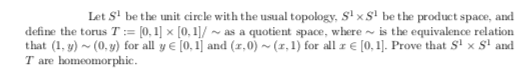Let S1 be the unit circle with the usual topology, S1 × S1 be the product space, and define the torus T : = [0,1] × [0,1] / ∼ as a quotient space, where ∼ is the equivalence relation that (1,y) ∼ (0,y) for all y ∈ [0,1] and (x,0) ∼ (x,1) for all x ∈ [0,1]. Prove that S1 × S1 and T are homeomorphic.

Let Sl be the unit circle with the usual topology, Stx St be the product space, and define the torus T := [0,1] [0,1]/ -as a quotient space, where is the equivalence relation that (1, y) (0,y) for all y € (0,1) and (1,0) (1,1) for all € [0,1]. Prove that Six Stand T are homeomorphic.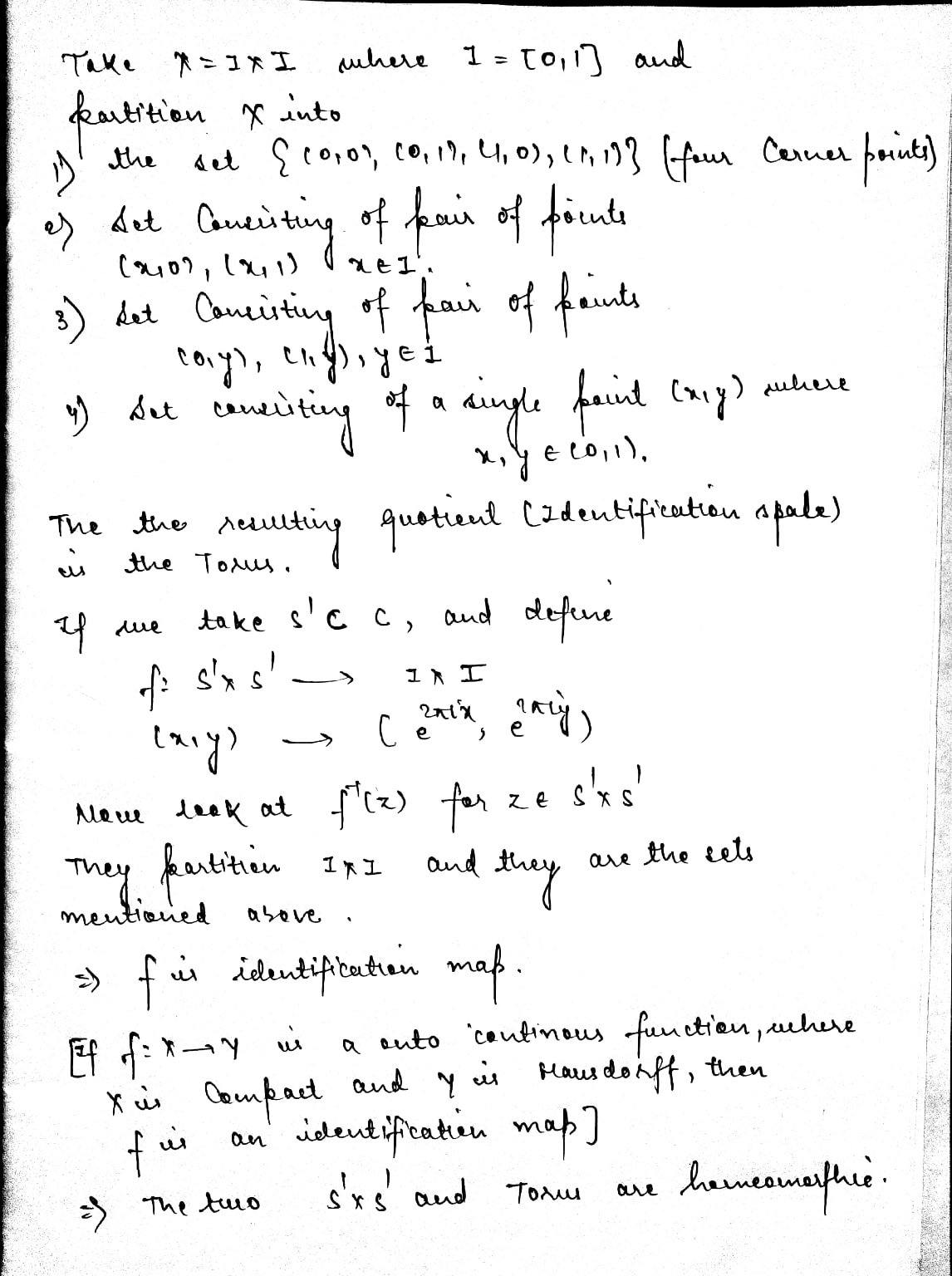##### Add Answer of: Let S1 be the unit circle with the usual topology, S1 × S1 be the product...
Similar Homework Help Questions
• ### Let S 1 be the unit circle with the usual topology, S 1×S 1 be the...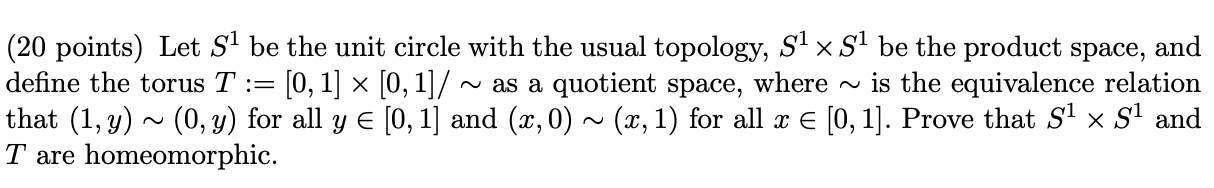Let S 1 be the unit circle with the usual topology, S 1×S 1 be the product space, and define the torus T := [0, 1] × [0, 1]/ ∼ as a quotient space, where ∼ is the equivalence relation that (1, y) ∼ (0, y) for all y ∈ [0, 1] and (x, 0) ∼ (x, 1) for all x ∈ [0, 1]. Prove that S 1 × S 1 and T are homeomorphic. (20 points) Let Sl be...

• ### 3. (20 points) Let Sl be the unit circle with the usual topology, Six Sl be...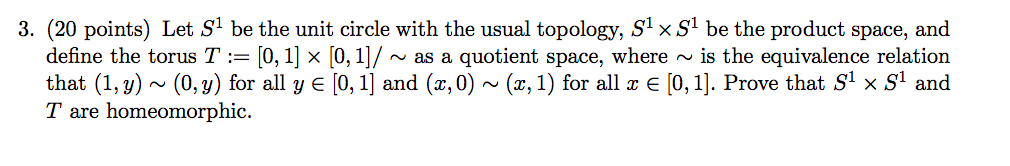3. (20 points) Let Sl be the unit circle with the usual topology, Six Sl be the product space, and define the torus T := [0, 1] x [0, 1]/ ~ as a quotient space, where ~ is the equivalence relation that (1, y) ~ (0,y) for all y € [0, 1] and (x, 0) ~ (x,1) for all x € [0, 1]. Prove that S1 x S1 and T are homeomorphic.

• ### Consider R with the usual Euclidean topology and let I = [0, 1] be the closed...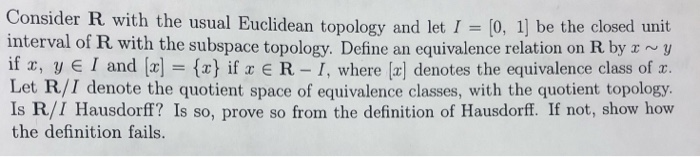Consider R with the usual Euclidean topology and let I = [0, 1] be the closed unit interval of R with the subspace topology. Define an equivalence relation on R by r ~y if x, y E I and [x] = {x} if x € R – I, where [æ] denotes the equivalence class of x. Let R/I denote the quotient space of equivalence classes, with the quotient topology. Is R/I Hausdorff? Is so, prove so from the definition of...

• ### Let S2 denote the 2-dimensional sphere. Define the complex projective line 1 as the quotient space...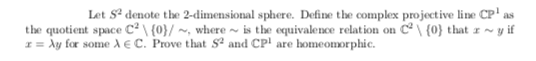Let S2 denote the 2-dimensional sphere. Define the complex projective line 1 as the quotient space 2 \ {0} / ∼ , where ∼ is the equivalence relation on 2 \ {0} that x ∼ y if x = λy for some λ∈C. Prove that S2 and 1 are homeomorphic. Let S denote the 2-dimensional sphere. Define the complex projective line CP as the quotient space C {0}/~, where is the equivalence relation on {0} that I ~y if r...

• ### 5. (20 points) Let S denote the 2-dimensional sphere. Define the complex projective line CP as...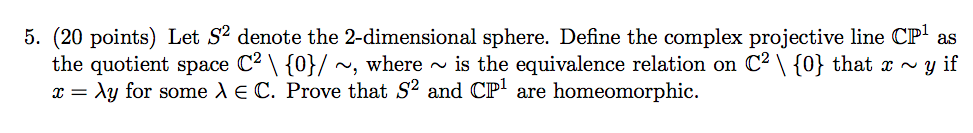5. (20 points) Let S denote the 2-dimensional sphere. Define the complex projective line CP as the quotient space C2 \ {0}/ ~, where ~ is the equivalence relation on C2 \ {0} that æ ~y if x = ly for some de C. Prove that s2 and CPl are homeomorphic.

• ### Topology 3. Either prove or disprove each of the following statements: (a) If d and p...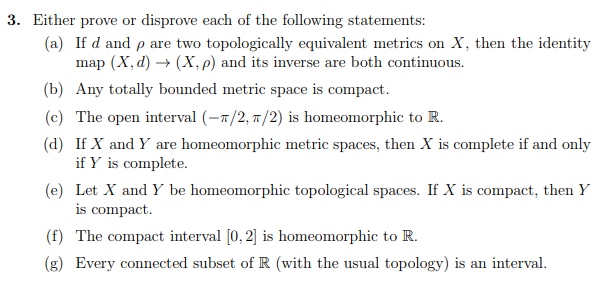Topology 3. Either prove or disprove each of the following statements: (a) If d and p map (X, d) X, then the identity topologically equivalent metrics (X, p) and its inverse are both continuous are two on (b) Any totally bounded metric space is compact. (c) The open interval (-r/2, n/2) is homeomorphic to R (d) If X and Y are homeomorphic metric spaces, then X is complete if and only if Y is complete (e) Let X and Y...

• ### Let X = {(x, y) ∈ R2: x ≥ 0 or y = 0}; and let τ be the subspace topology on X induced by the usu...

Let X = {(x, y) ∈ R2: x ≥ 0 or y = 0}; and let τ be the subspace topology on X induced by the usual topology on R2 . Suppose R has the usual topology and we define f : X → R by f((x, y)) = x for each (x, y) ∈ X. Show that f is a quotient map, but it is neither open nor closed.(So, a restricted function of an open function need not be...

• ### 3. (a) Let (R, τe) be the usual topology on R. Find the limit point set...

3. (a) Let (R, τe) be the usual topology on R. Find the limit point set of the following subsets of R (i) A = { n+1 n : n ∈ N} (ii) B = (0, 1] (iii) C = {x : x ∈ (0, 1), x is a rational number (b) Let X denote the indiscrete topology. Find the limit point set A 0 of any subset A of X. (c) Prove that a subset D of X is...

• ### L maps is a quotient map. 4, Let ( X,T ) be a topological space, let Y be a nonempty set, let f b...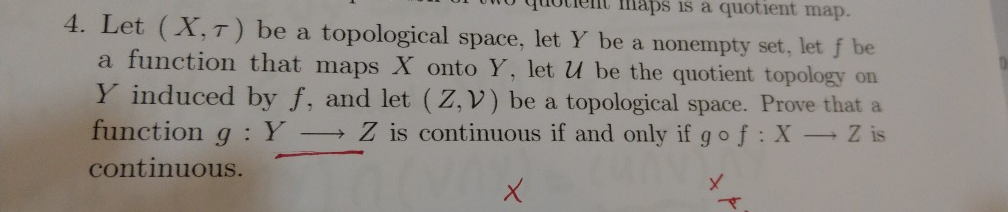l maps is a quotient map. 4, Let ( X,T ) be a topological space, let Y be a nonempty set, let f be a function that maps X onto Y, let U be the quotient topology on induced by f, and let (Z, V) be a topological space. Prove that a function g:Y Z is continuous if and only if go f XZ is continuous. l maps is a quotient map. 4, Let ( X,T ) be a topological...

• ### Let X and Y be topological spaces, and let X × y be equipped with the product topology. Let yo E Y be fixed. Define the map f XXx Y by f(x) (x, yo) Prove that f is continuous, Let X and Y be top...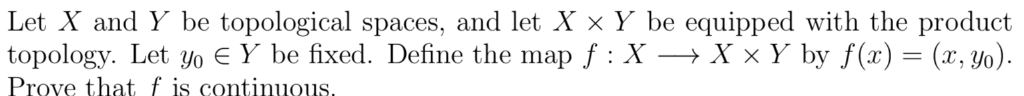Let X and Y be topological spaces, and let X × y be equipped with the product topology. Let yo E Y be fixed. Define the map f XXx Y by f(x) (x, yo) Prove that f is continuous, Let X and Y be topological spaces, and let X × y be equipped with the product topology. Let yo E Y be fixed. Define the map f XXx Y by f(x) (x, yo) Prove that f is continuous,

Free Homework App